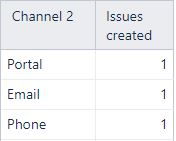# Custom Calculated member in Channel Dimension

Hello,
I need to retrieve Channel statistics. There is a Service Desk project and it has Email and Portal as Channel. However, I need to divide Portal into more dimensions. I would write CASE WHEN formula in Channel Dimension. Something like this:

CASE
WHEN [Measures].[Issue Channel] = ‘Email’
THEN ‘Email’
WHEN [Measures].[Issue Channel] = ‘Jira’
THEN ‘Jira’
WHEN [Measures].[Issue Channel] = ‘Portal’ AND [Measures].[Issue Customer Request Type] = ‘HR Internal Open request’
THEN ‘Phone’
ELSE ‘Portal’
END

This example is from Measures, but its not useful for me. All I need is a list of Channels and no. of issues next to each of them. Like this:Is this possible to do at all?

Thank you

Hi @Osvalda

Make sure that you have selected and imported both fields “Channel” and “Curstomer Request type” as dimensions in eazyBI.

Then select the “Channel” dimension in report rows and expand to see each channel as separate row.
Later remove the “All channels” row from the report.
Then create a new calculated member with the name “Phone” in the “Channel” dimension and use the formula

`NULL`

Then select the calculated member in your report.

Now create a new user-defined calculated measure in “Measures” dimension using this formula:

``````CASE
WHEN
[Channel].CurrentMember.Name = "Email"
OR
[Channel].CurrentMember.Name = "Jira"
THEN
Nonzero((
[Measures].[Issues created]
))
WHEN
[Channel].CurrentMember.Name = "Phone"
THEN
Nonzero((
[Measures].[Issues created],
[Channel].[Portal],
[Customer Request type].[HR]
))
WHEN
[Channel].CurrentMember.Name = "Portal"
THEN
Nonzero((
[Measures].[Issues created],
[Channel].[Portal]
))
-
Nonzero((
[Measures].[Issues created],
[Channel].[Portal],
[Customer Request type].[HR]
))
END
``````

Martins / eazyBI support

1 Like